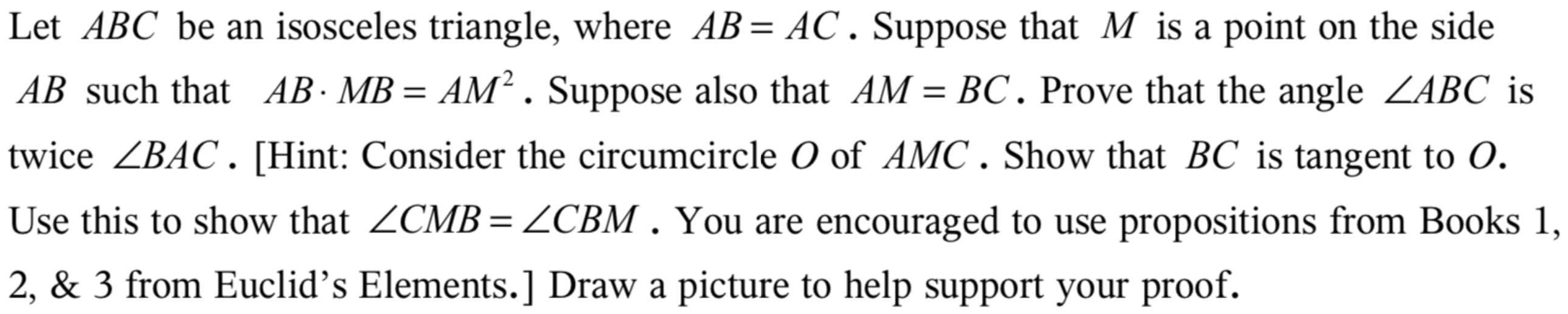# |Let ABC be an isosceles triangle, where AB= AC. Suppose that M is a point on the sideAB such that AB MB = AM2 . Suppose also that AM BC. Prove that the angle ZABC istwice ZBAC. [Hint: Consider the circumcircle O of AMC. Show that BC is tangent to OUse this to show that ZCMB= ZCBM . You are encouraged to use propositions from Books 1| 2, & 3 from Euclid's Elements.] Draw a picture to help support your proof.

Question
125 viewshelp_outlineImage Transcriptionclose|Let ABC be an isosceles triangle, where AB= AC. Suppose that M is a point on the side AB such that AB MB = AM2 . Suppose also that AM BC. Prove that the angle ZABC is twice ZBAC. [Hint: Consider the circumcircle O of AMC. Show that BC is tangent to O Use this to show that ZCMB= ZCBM . You are encouraged to use propositions from Books 1 | 2, & 3 from Euclid's Elements.] Draw a picture to help support your proof. fullscreen
check_circle

Step 1

To show that (under the given conditions) that angle A = 36 and (hence B=72=2A)

Step 2

We may take AB = AC =1 and AM = BC=x. The given condition yields a quadratic equation for x.

Step 3

Solve for x.&...

### Want to see the full answer?

See Solution

#### Want to see this answer and more?

Solutions are written by subject experts who are available 24/7. Questions are typically answered within 1 hour.*

See Solution
*Response times may vary by subject and question.
Tagged in

### Math